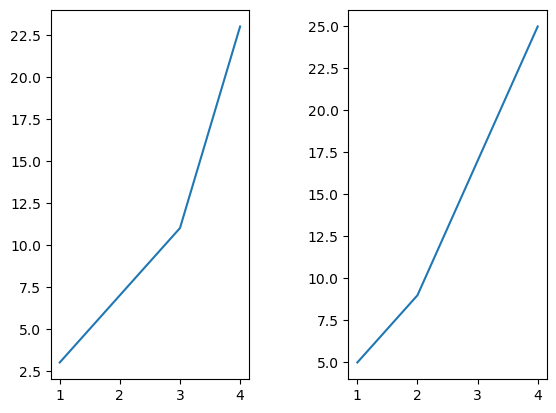# # How to export one image with multiple plots with matplotlib

Just as we did previously to create multiple plots using `add_subplot()`, we can export the finalised figure after:

``````import matplotlib.pyplot as plt

figure = plt.figure()

ax1.plot([1, 2, 3, 4], [3, 7, 11, 23])
ax2.plot([1, 2, 3, 4], [5, 9, 17, 25])

figure.savefig("graphs.png")
``````

This produces simple output using the default `subplots_adjust()` configuration:We can adjust the spacing between plots using `subplots_adjust()`:

``````import matplotlib.pyplot as plt

figure = plt.figure()

ax1.plot([1, 2, 3, 4], [3, 7, 11, 23])
ax2.plot([1, 2, 3, 4], [5, 9, 17, 25])

figure.savefig("graphs.png")
``````

Now the axes are separated by 50% of the average x axis width:We can set `bbox_inches="tight"` to constrain the plots as tightly as possible (while still leaving the configured `wspace`). Remember the default padding around the side of the figure is `0.1`:

``````import matplotlib.pyplot as plt

figure = plt.figure()

ax1.plot([1, 2, 3, 4], [3, 7, 11, 23])
ax2.plot([1, 2, 3, 4], [5, 9, 17, 25])

figure.savefig("graphs.png", bbox_inches="tight")
``````

This shrinks the borders around the axes:## # Recap

To recap, the `.savefig()` arguments change the size of the figure. You can set it to constrain the plots inside it, and you can also add padding.

The `subplots_adjust()` arguments change each of the plot settings.